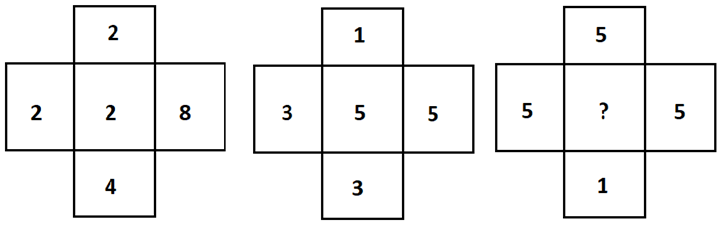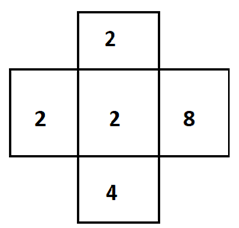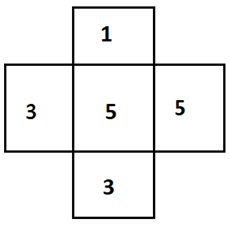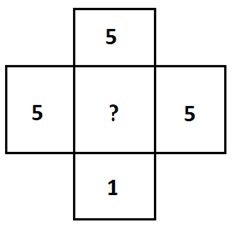Question of The Day25-06-2020

In the following question, select the number which can be placed at the sign of question mark from the given alternative.Correct Answer : a ) 5

Explanation :

Here, the common logic is that,

The middle number can be obtained by dividing the product of numbers in a row with the product of numbers in a column excluding the middle number.

Considering the first figureThus, here

$$2={2*8 \over 2*4}$$

Similarly, for the second figureThus, here

$$5={3*5 \over 1*3}$$

Thus, for the final figure our missing term will beMissing term=$${5*5 \over 1*5}=5$$

Hence, (a) is the correct answer.

Such type of question is asked in various government exams like SSC CGL, SSC MTS, SSC CPO, SSC CHSL, RRB JE, RRB NTPC etc.

Read Daily Current Affairs, Banking Awareness, Hindi Current Affairs, Word of the Day, and attempt free mock tests at PendulumEdu and boost your preparation for the actual exam.0